# Data Driven Logo Design with ggraph and gtable

## Oct 7, 2016 · 1563 words · 8 minutes read R ggraph design

After having “succesfully” launched my blog I felt the time had come to create some identity for it (and by extension, me). What better way to do this than create a logo? This thought didn’t suddenly appear in my mind - if you’ve read the announcement post you will now that such details have been rummaging around my head for as long as the thought of a blog.

My initial idea was to make something very stylistic in the vein of a d i ligature in a classic serif font - I have absolutely no idea where the thought came from, but I think it was inspired by writing my PhD dissertation with the EB Garamond font. Something about the idea didn’t click though: Data Imaginist and then a typography-based logo? No, it had to convey a sense of playfulness with data and visualization! I still wanted a D and an I somehow, so the question ended up with how to create data visualizations in the shape of letters.

## Drawing a D

My instinct with the D was to make a sort of horizontal bar chart that roughly outlined the shape of a D. I kind of felt that this was a bit too boring and unimaginative though, so I continued to look. Something got my thinking of an arc diagram and how the correct graph structure could actually produce a pretty decent D, with a hole and everything. Such a graph structure would be hard to find in the wild though so I had to make one myself.

``````# Create a random graph with 100 nodes and 1000 edges
d_data <- data.frame(from = sample(100, 1000, T), to = sample(100, 1000, T))
# Remove all loops
d_data <- d_data[d_data\$from != d_data\$to, ]
# Find all edges that connects the top and bottom (the large arc)
d_data_top <- d_data[abs(d_data\$from - d_data\$to) >= 75, ]
# Find a subset of edges that only connects nodes close to each other (the stem)
d_data_bottom <- d_data[sample(which(abs(d_data\$from - d_data\$to) <= 25), 200), ]
# Assemble it all to an igraph object
d_graph <- graph_from_edgelist(as.matrix(unique(rbind(d_data_top, d_data_bottom))))
# Add a random class to each node
V(d_graph)\$class <- sample(letters[1:10], length(unique(unlist(d_data))), T)``````

Bear in mind that a lot of parameter tweaking went into finding a nice looking graph. Also, keep in mind that `sample` is used in a few places so if you run this you will end up with a slightly different graph. I never recorded the seed I used when I created the logo so my version will forever be unique.

With the data ready it was time to draw the D. Thankfully I had already implemented an arc diagram layout in ggraph so it should be a piece of cake. In order to add some flair to the plot I decided to draw the arcs with an opacity gradient from start to end:

``````D <- ggraph(d_graph, layout = 'linear') +
geom_edge_arc(aes(x=y, y=x, xend=yend, yend=xend, alpha = ..index..,
color = node2.class))
D``````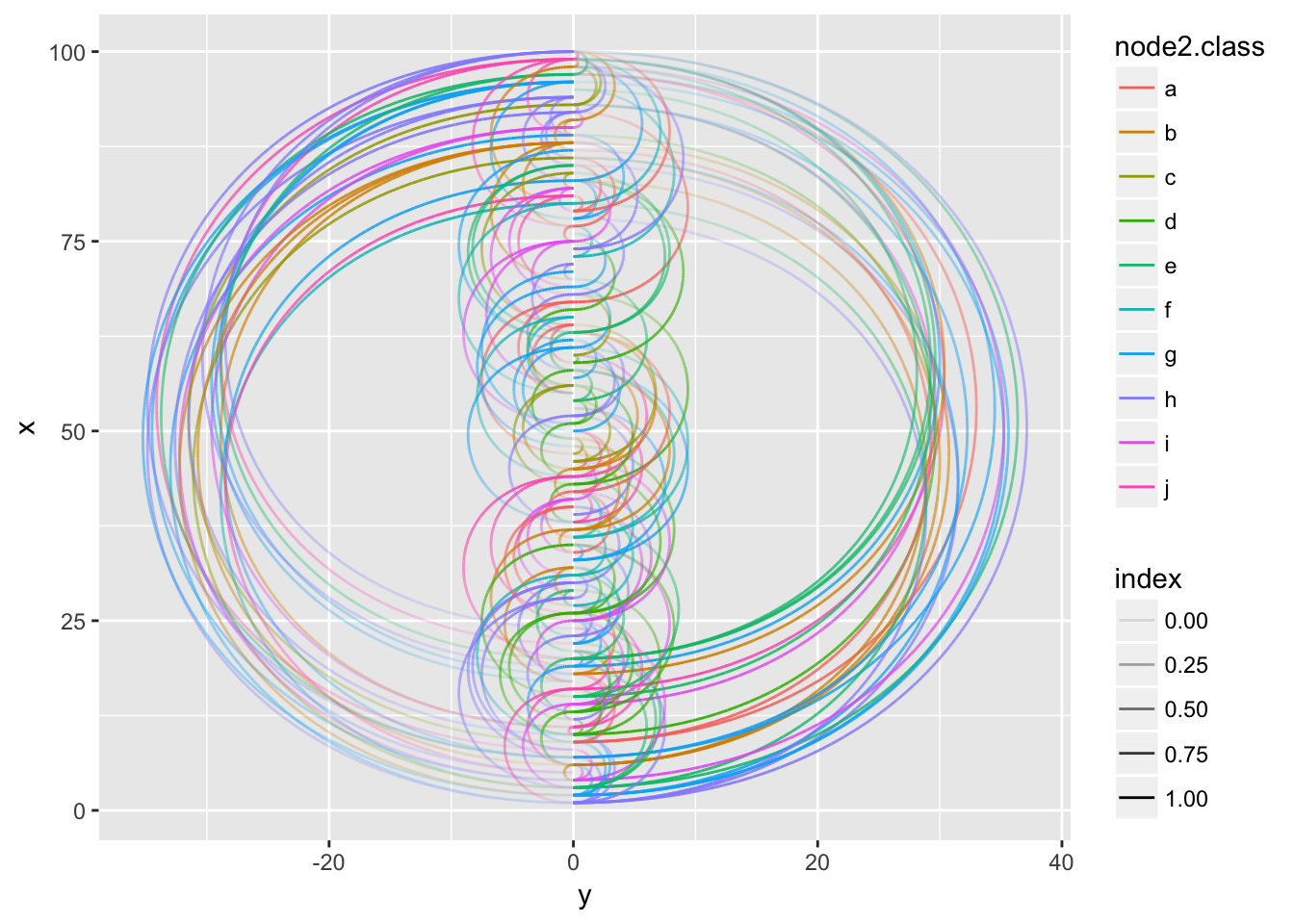Hmm, there is the idea of a D somewhere in that plot but it was not what I had envisioned. The “problem” is that edges going in different directions are positioned on different sides of the y-axis. I could of course change the graph so all edges went in the same direction, but then the gradient would also go in the same direction for each arc, which was not what I wanted. The nice thing about being the developer behind your own tools is that you can always make them do your biding, so I promptly added a `fold` argument to `geom_edge_arc()` that would put all arcs on the same side irrespectively of their direction (If you are ever to use this feature, be thankful that I had to draw a D).

``````D <- ggraph(d_graph, layout = 'linear') +
geom_edge_arc(aes(x=y, y=x, xend=yend, yend=xend, alpha = ..index..,
color = node2.class),
fold = TRUE)
D``````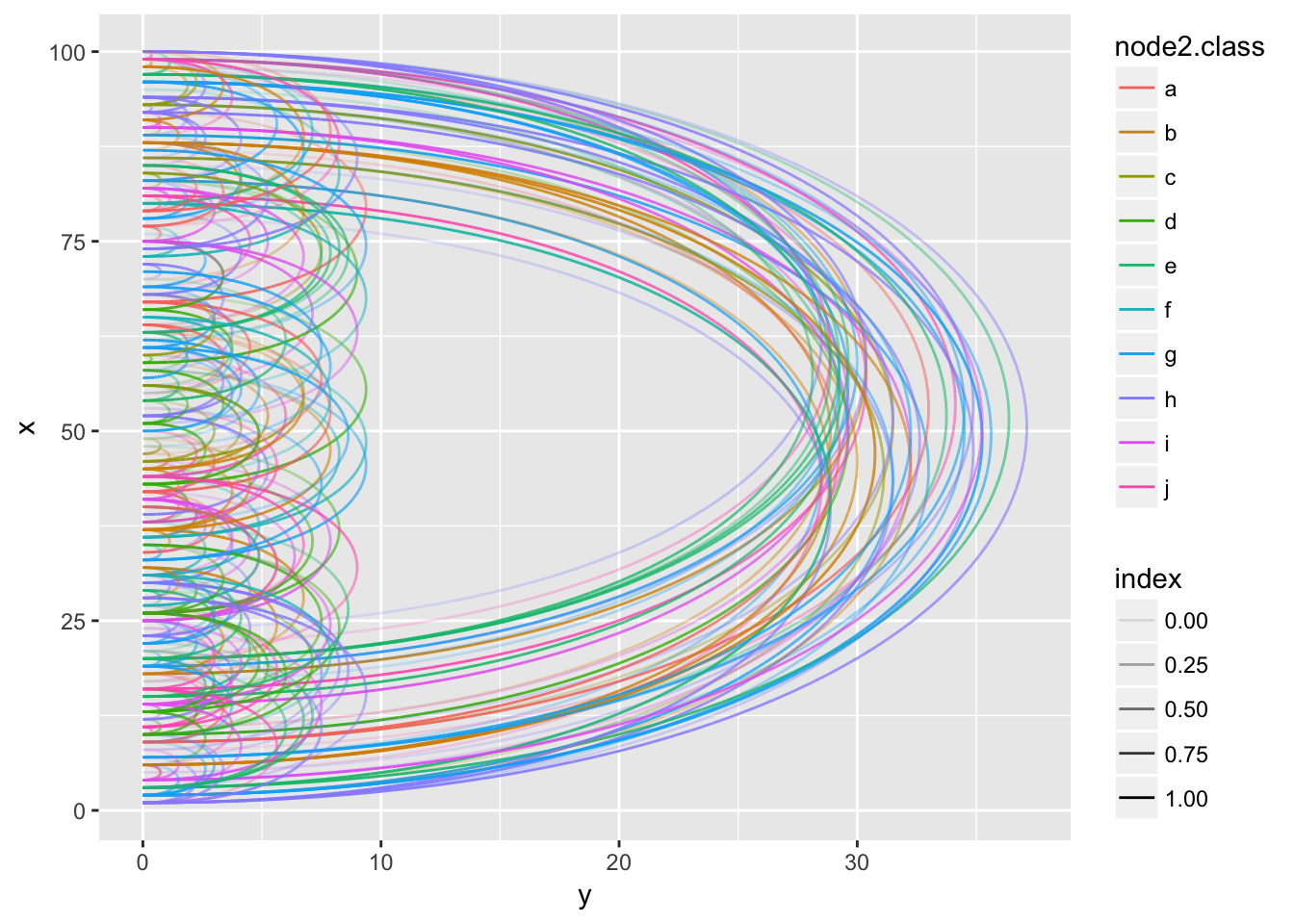Now we’re getting somewhere, but lets loose the legends and coordinate system and make the arcs a bit thicker while we’re at it…

``````theme_empty <- theme_void() +
theme(legend.position = 'none',
plot.margin = margin(0, 0, 0, 0, 'cm'),
legend.box.spacing = unit(0, 'cm'))

D <- ggraph(d_graph, layout = 'linear') +
geom_edge_arc(aes(x=y, y=x, xend=yend, yend=xend, alpha = ..index..,
color = node2.class),
fold = T, edge_width = 1) +
scale_y_continuous(expand = c(0.03,0)) +
theme_empty
D``````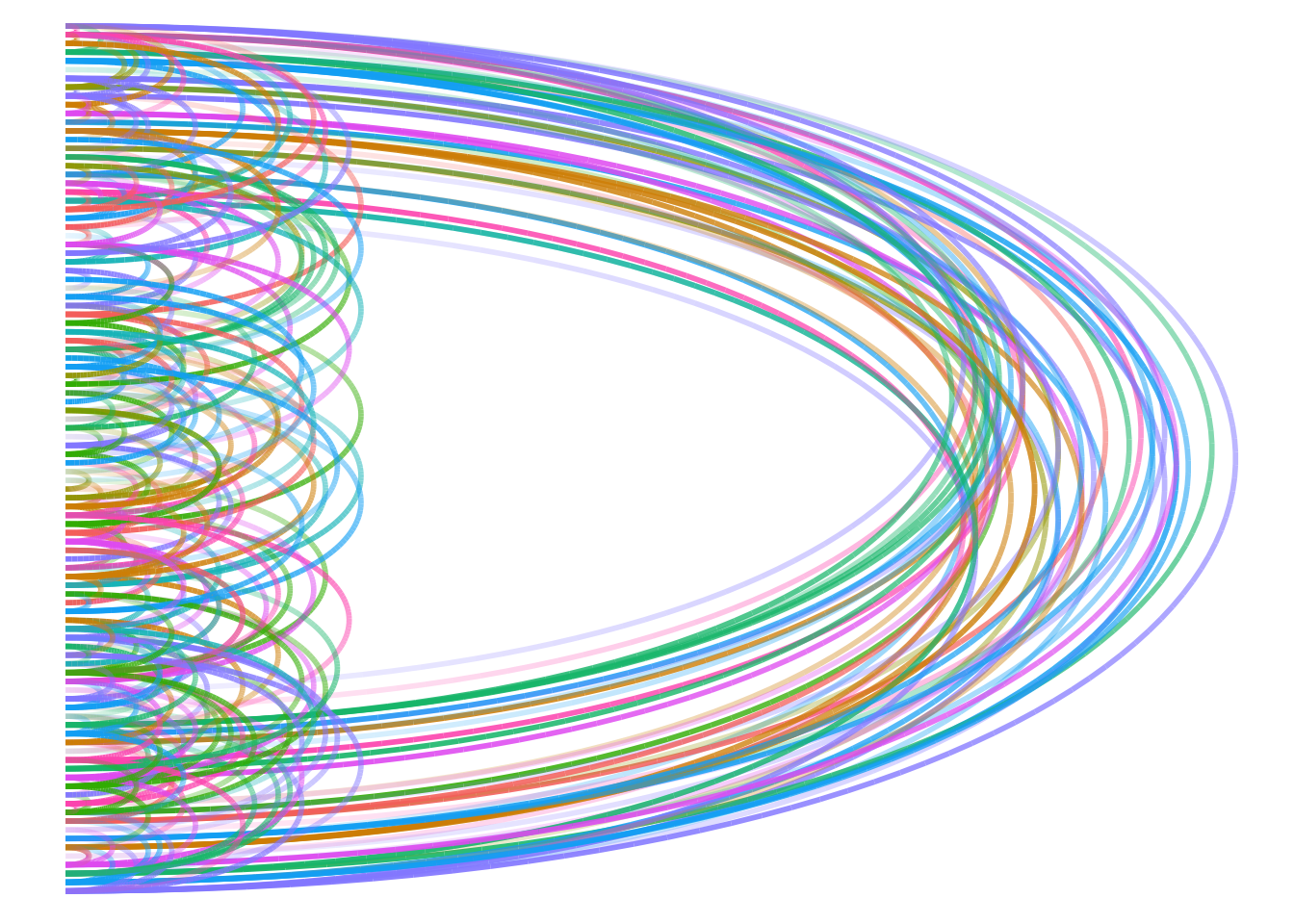# Drawing an I

The shape of an I is quite easier to make with a visualization, but it is in turn also more boring so the visualization would need to be a tad more interesting to make up for it. I decided to go for a lowercase i as it would allow my to do different things with both the dot and the stem. Already being in ggraph-land after the D I decided to continue down that road. A treemap would be a nice fit for the rectangular stem, while any circular layout would work well for the dot. The only thing to keep in mind for the treemap was that since it was meant to drawn as a very thin rectangle, the aspect ratio should be set in the layout to make sure the rectangles remains fairly square.

We don’t need to make up any data for the i as ggraph already comes with a hierarchical data structure we can use, namely the flare class hierarchy, so lets get right to it:

``````# Create an igraph object from the flare data
flareGraph <- graph_from_data_frame(flare\$edges, vertices = flare\$vertices)
# Set the class of each node to the name of their topmost-1 parent
flareGraph <- tree_apply(flareGraph, function(node, parent, depth, tree) {
tree <- set_vertex_attr(tree, 'depth', node, depth)
if (depth == 1) {
tree <- set_vertex_attr(tree, 'class', node, V(tree)\$shortName[node])
} else if (depth > 1) {
tree <- set_vertex_attr(tree, 'class', node, V(tree)\$class[parent])
}
tree
})
# Define wether a node is terminal
V(flareGraph)\$leaf <- degree(flareGraph, mode = 'out') == 0``````

With our graph at hand we can draw the stem:

``````i_stem <- ggraph(flareGraph, 'treemap', weight = 'size', width = 1, height = 3) +
geom_node_tile(aes(filter = leaf, fill = class, alpha = depth), colour = NA) +
geom_node_tile(aes(filter = depth != 0, size = depth), fill = NA) +
scale_alpha(range = c(1, 0.3), guide = 'none') +
scale_size(range = c(1.5, 0.4), guide = 'none') +
theme_empty
i_stem``````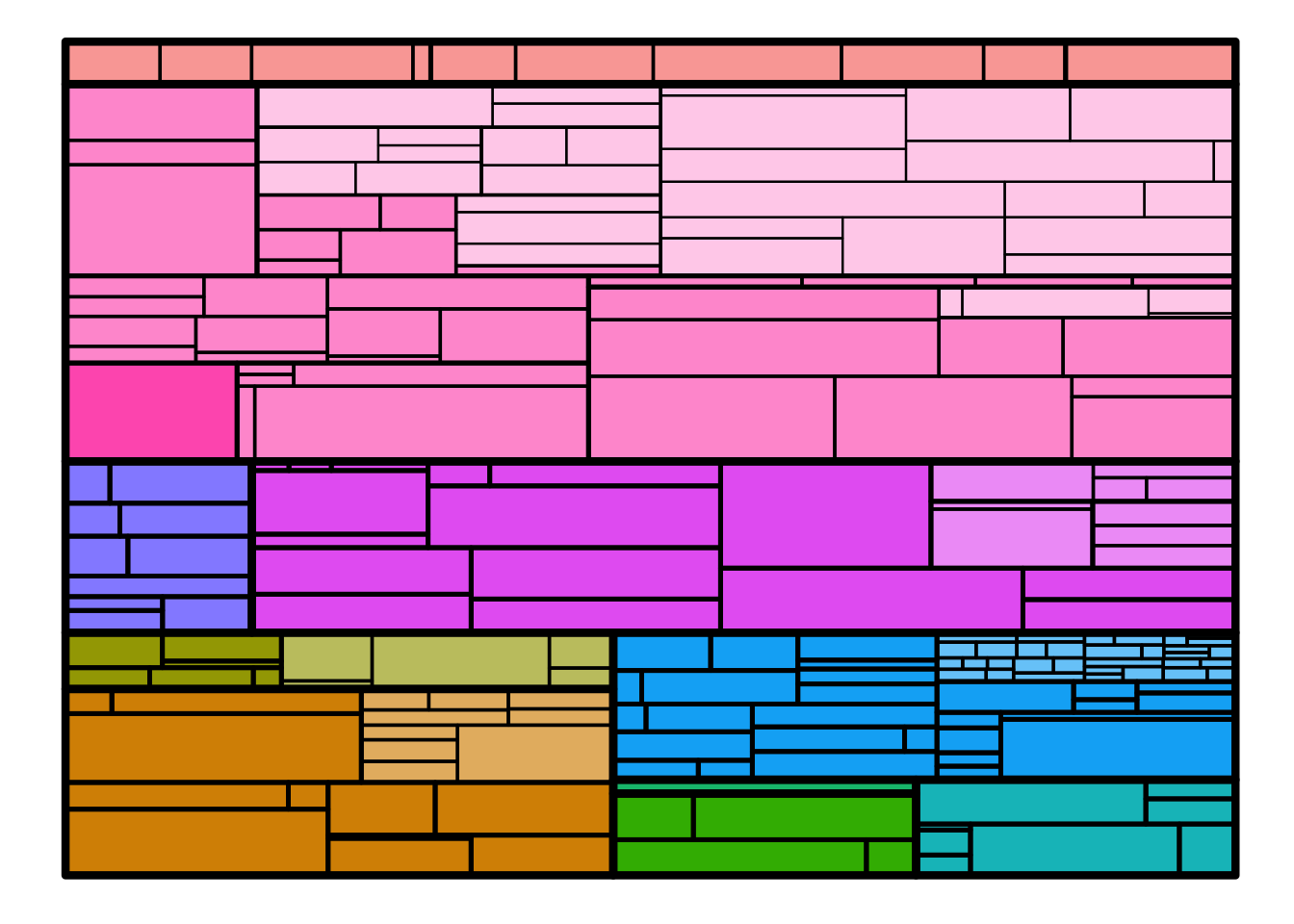Well, it looks decidedly non-thin, but this is just a matter of stretching it in the right direction…

For the dot we can continue with our flare graph and draw a circular hierarchy, but since we have same additional information about the flare data (which classes imports each other) we can do something more fancyfull by drawing these imports as hierarchical edge bundles. Hierarchical edge bundles is a way to bundle connections by letting them loosely follow an underlying hierarchy in the data structure and it makes for some very pretty plots.

``````importFrom <- match(flare\$imports\$from, flare\$vertices\$name)
importTo <- match(flare\$imports\$to, flare\$vertices\$name)
i_dot <- ggraph(flareGraph, 'dendrogram', circular = TRUE) +
geom_conn_bundle(aes(colour = ..index..), data = get_con(importFrom, importTo),
edge_alpha = 0.25) +
geom_node_point(aes(filter = leaf, colour = class)) +
coord_fixed() +
theme_empty
i_dot``````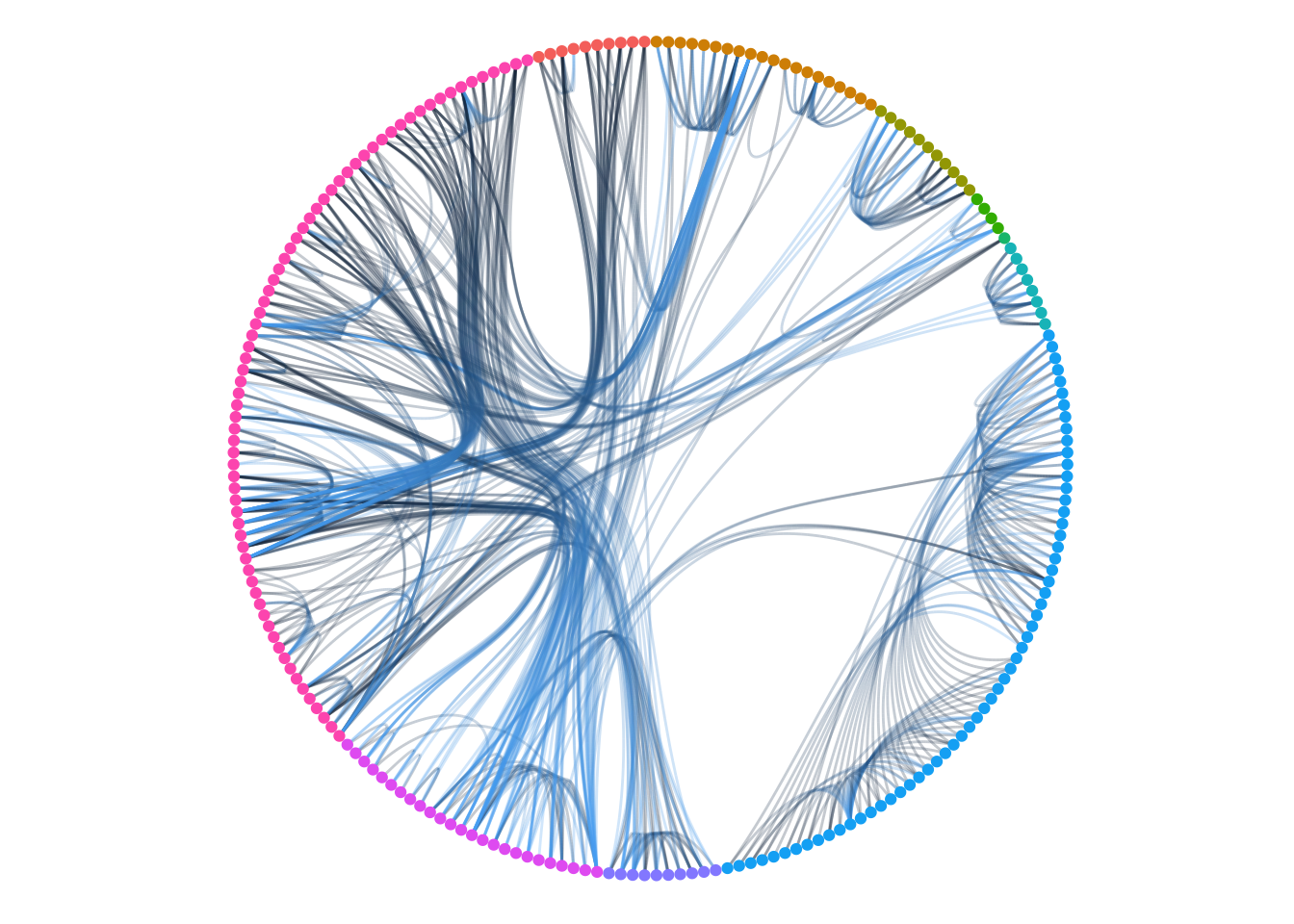What a nice dot!

The last touch before we assemble it all is to apply a less ggplot2-y colour scale. I have absolutely no perfect method to this. I like to scavenge places like Adobe Color CC but these palettes are mainly for design work and often only provides <=5 different colours (which is often too few for data visualizations). This time I decided to expand on one of the palettes (Flat design colors 1) by adding darker copies of each color to it. In the end the palette ended up like this

``````palette <- paste0('#', c('2B6E61', 'AB9036', '99532B', '9C3F33', '334D5C',
'45B29D', 'EFC94C', 'E27A3F', 'DF5A49', '677E52'))

D <- D + scale_edge_color_manual(values = palette)
i_stem <- i_stem + scale_fill_manual(values = palette)
i_dot <- i_dot + scale_edge_colour_gradient('', low = 'white', high = '#2C3E50') +
scale_color_manual(values = palette)
print(D)
print(i_stem)
print(i_dot)``````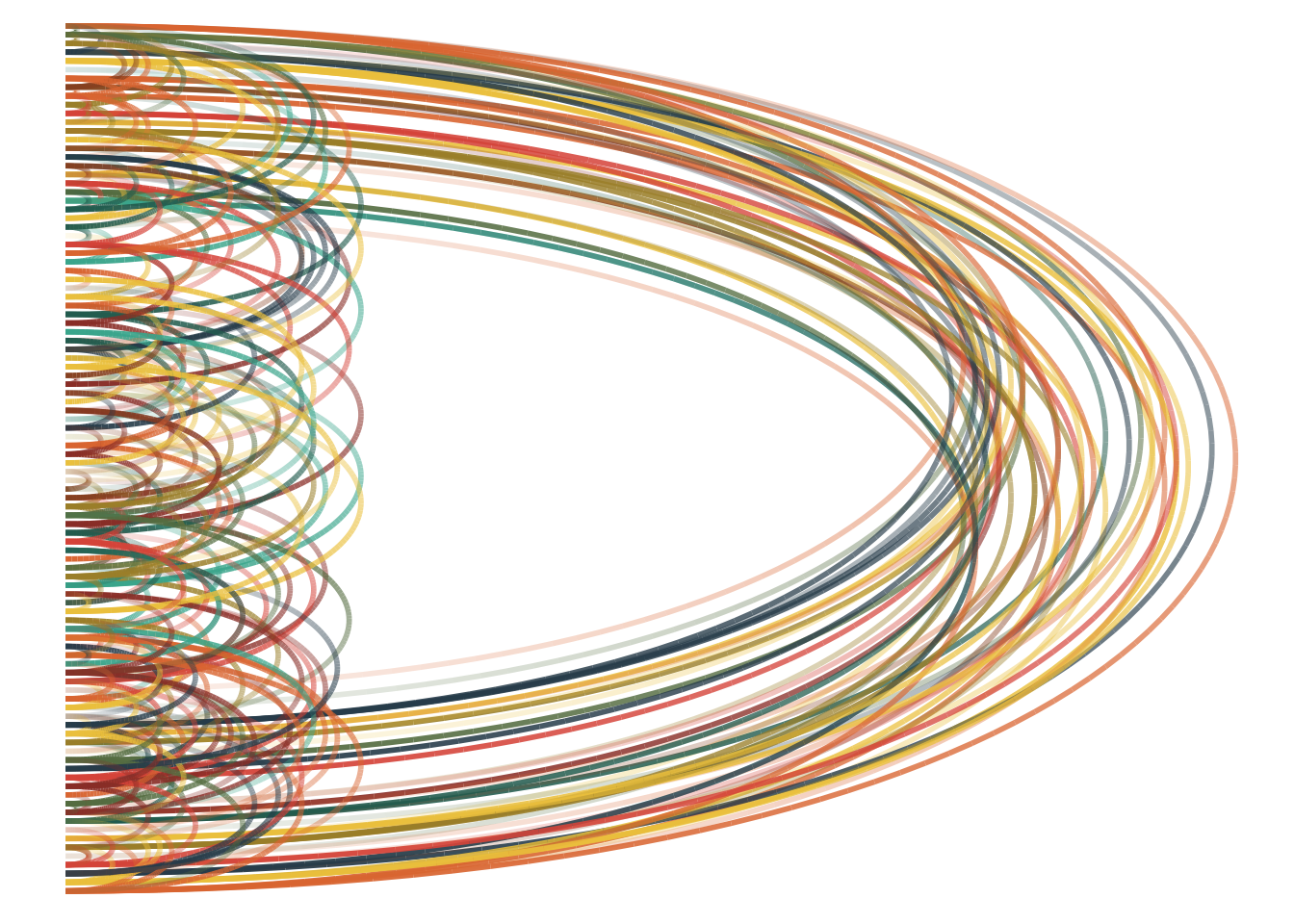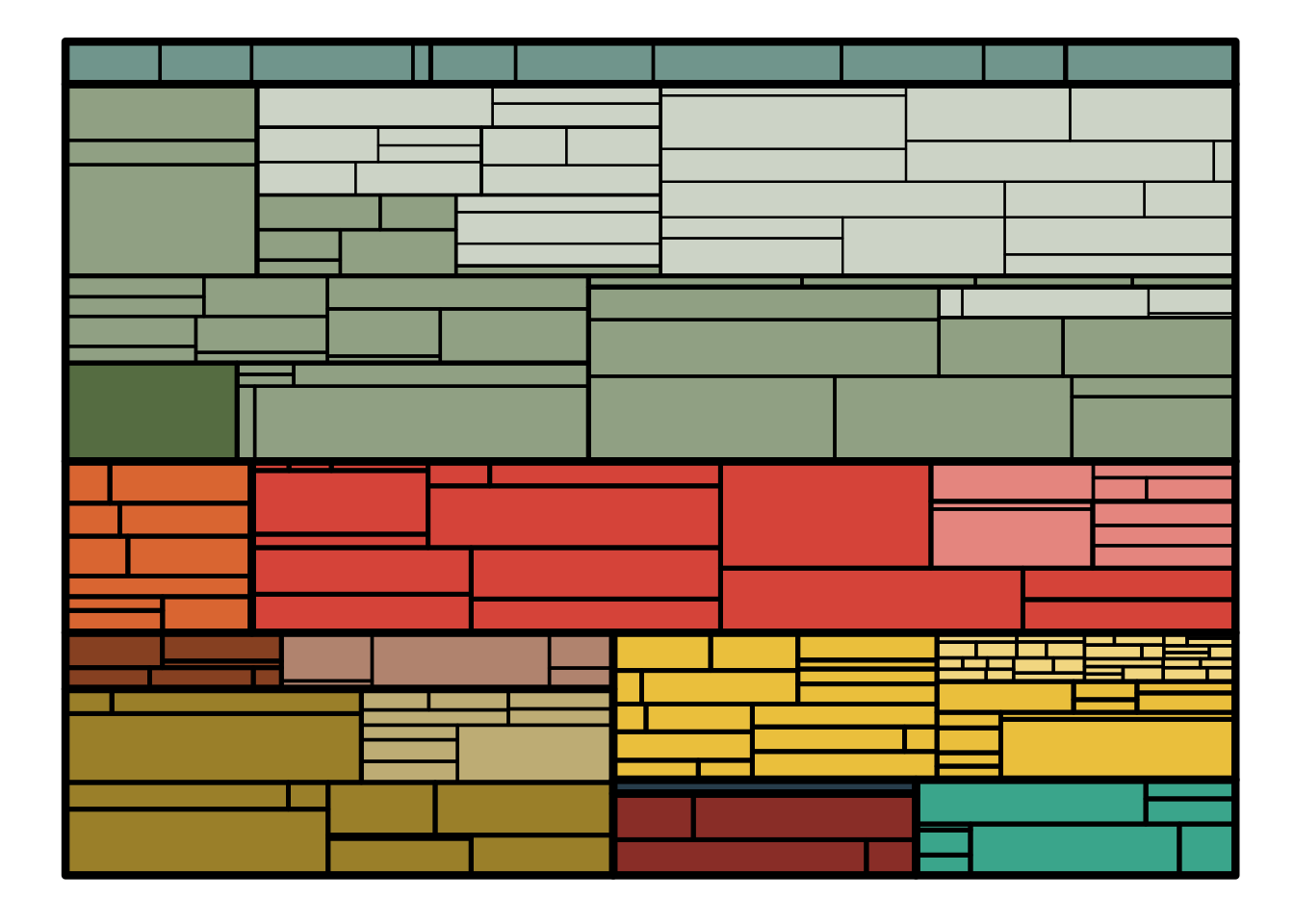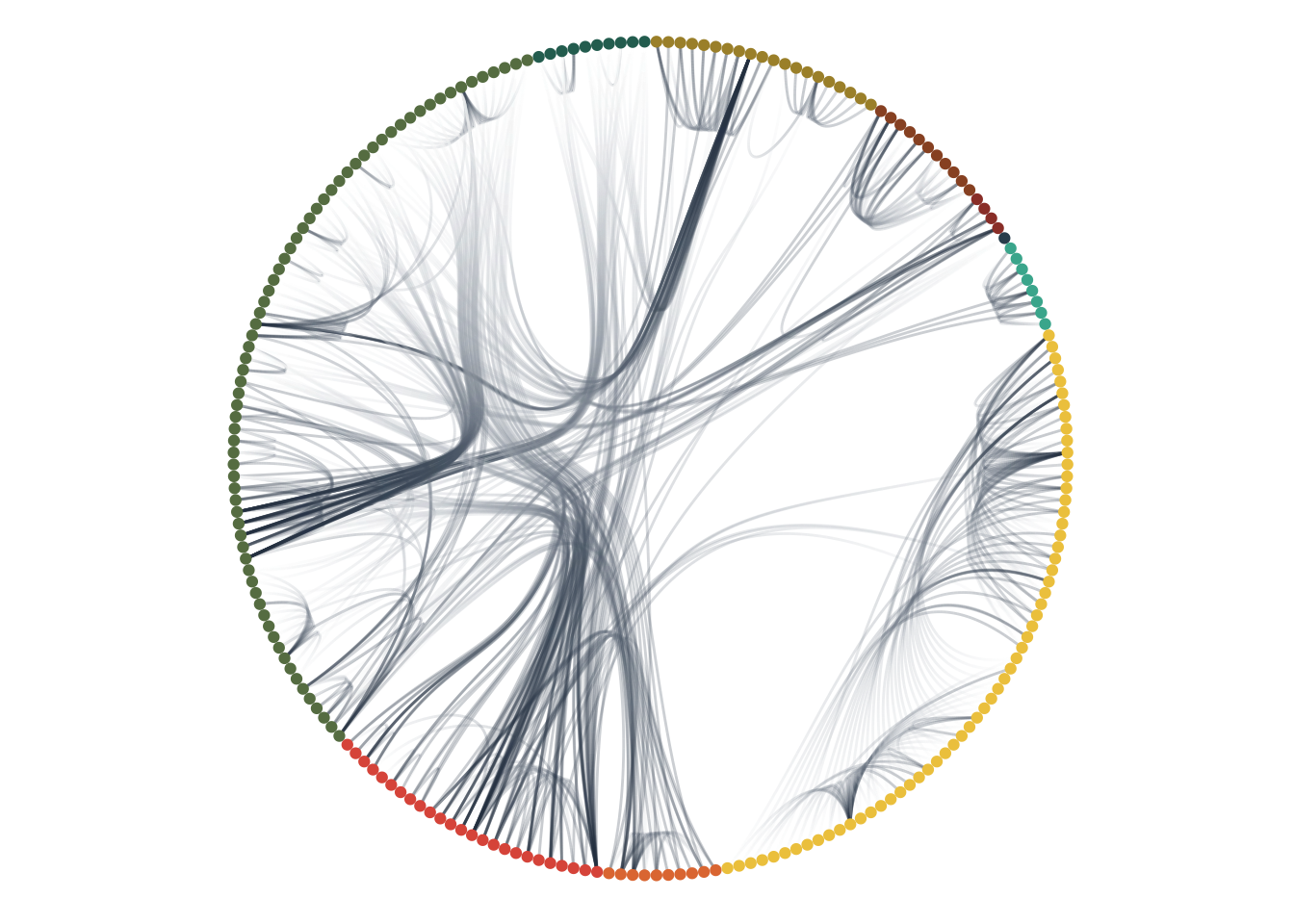# The Assembly

gtable is an underappreciated part of the whole ggplot2 experience as user rarely know that it is this layout engine that is used to position all those nice geoms. While it is rarely used outside of ggplot2 it does not have to be so.

At its simplest gtable is a way to define a grid with varying widths and heights of each column/row and add content that spans one or multiple rows/columns. For our logo we want a wide column for the D and a thinner column for the i as well as some spacing. The dot needs to be placed in a square cell so we need to have a row with the same height as the width of the i column. In the end we (through some trial and error) ends up with this grid: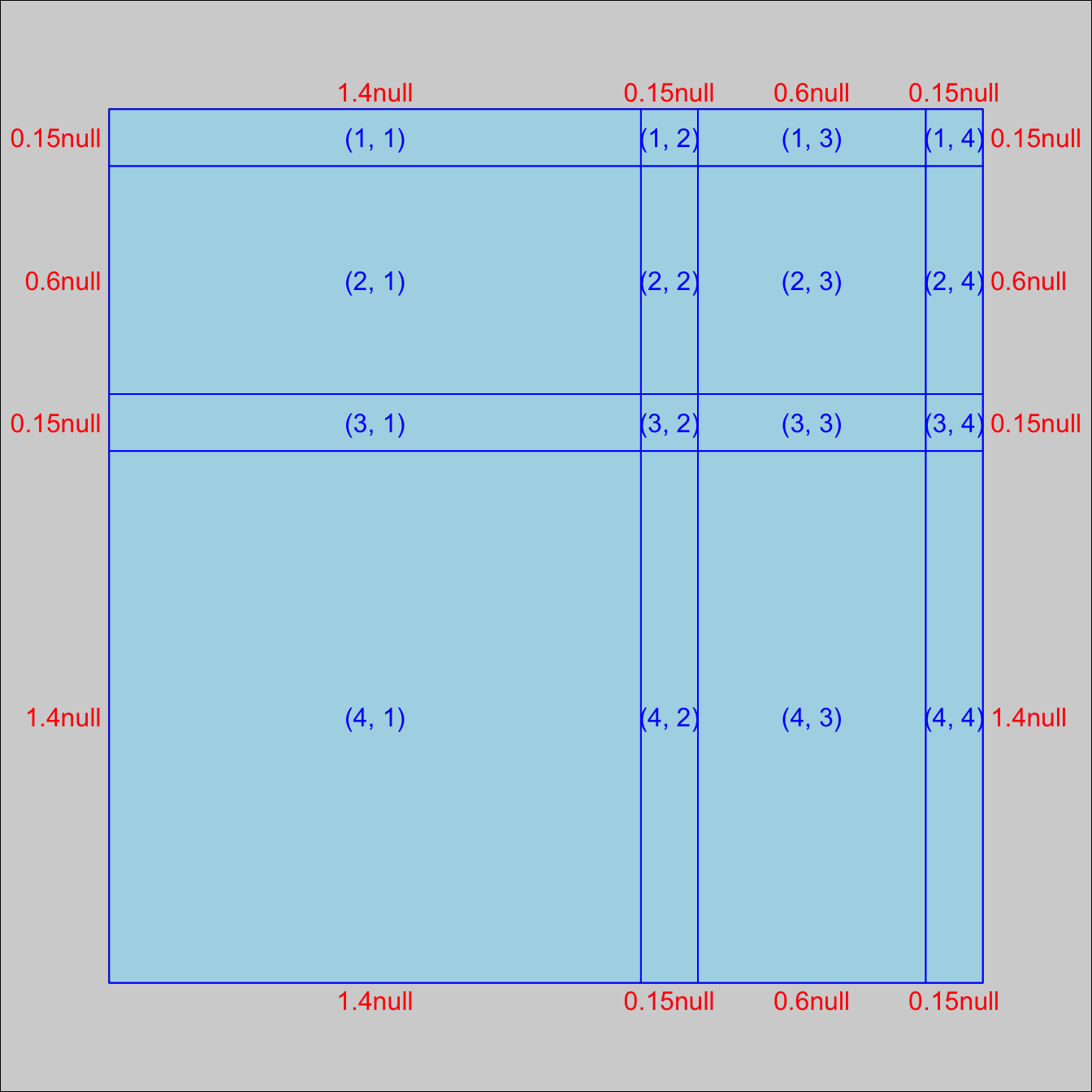Before we can put our letters into the grid, they need to be converted into something that gtable (or grid) can understand. Thankfully ggplot2 exports this functionality in the form of the `ggplotGrob()` function.

``````D_table <- ggplotGrob(D)
i_dot_table <- ggplotGrob(i_dot)
i_stem_table <- ggplotGrob(i_stem)
composite <- gtable(widths = unit(c(1.4, 0.15, 0.6, 0.15), 'null'),
heights = unit(c(0.15, 0.6, 0.15, 1.4), 'null'),
respect = TRUE)
composite <- gtable_add_grob(composite, D_table, 1, 1, 4, 2)
composite <- gtable_add_grob(composite, i_dot_table, 1, 2, 3, 4)
composite <- gtable_add_grob(composite, i_stem_table, 4, 3)
grid.newpage()
grid.draw(composite)``````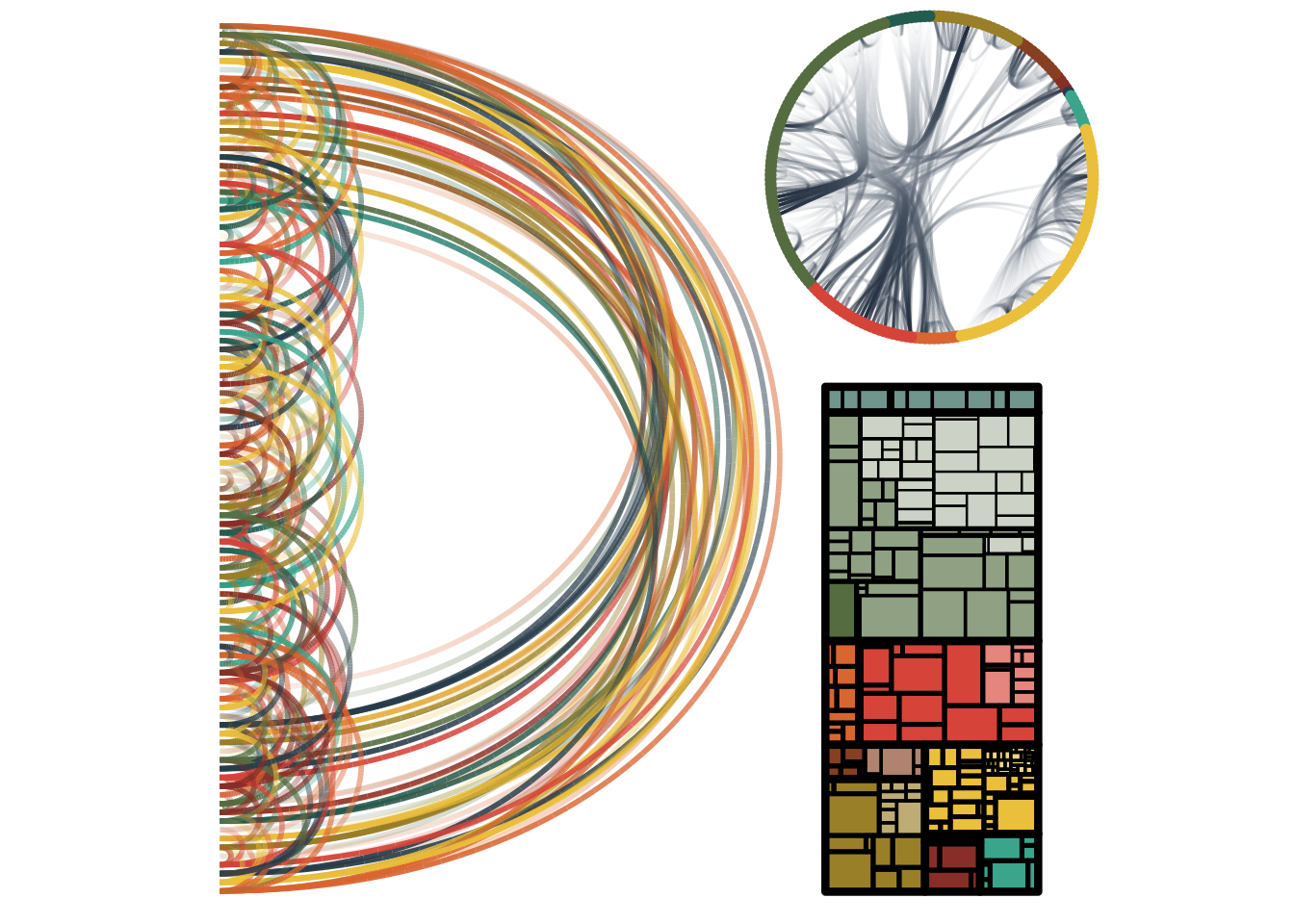É viola! A (sort of) reproducible logo made entirely in R. In a future post I will investigate how we can add some animation to it…